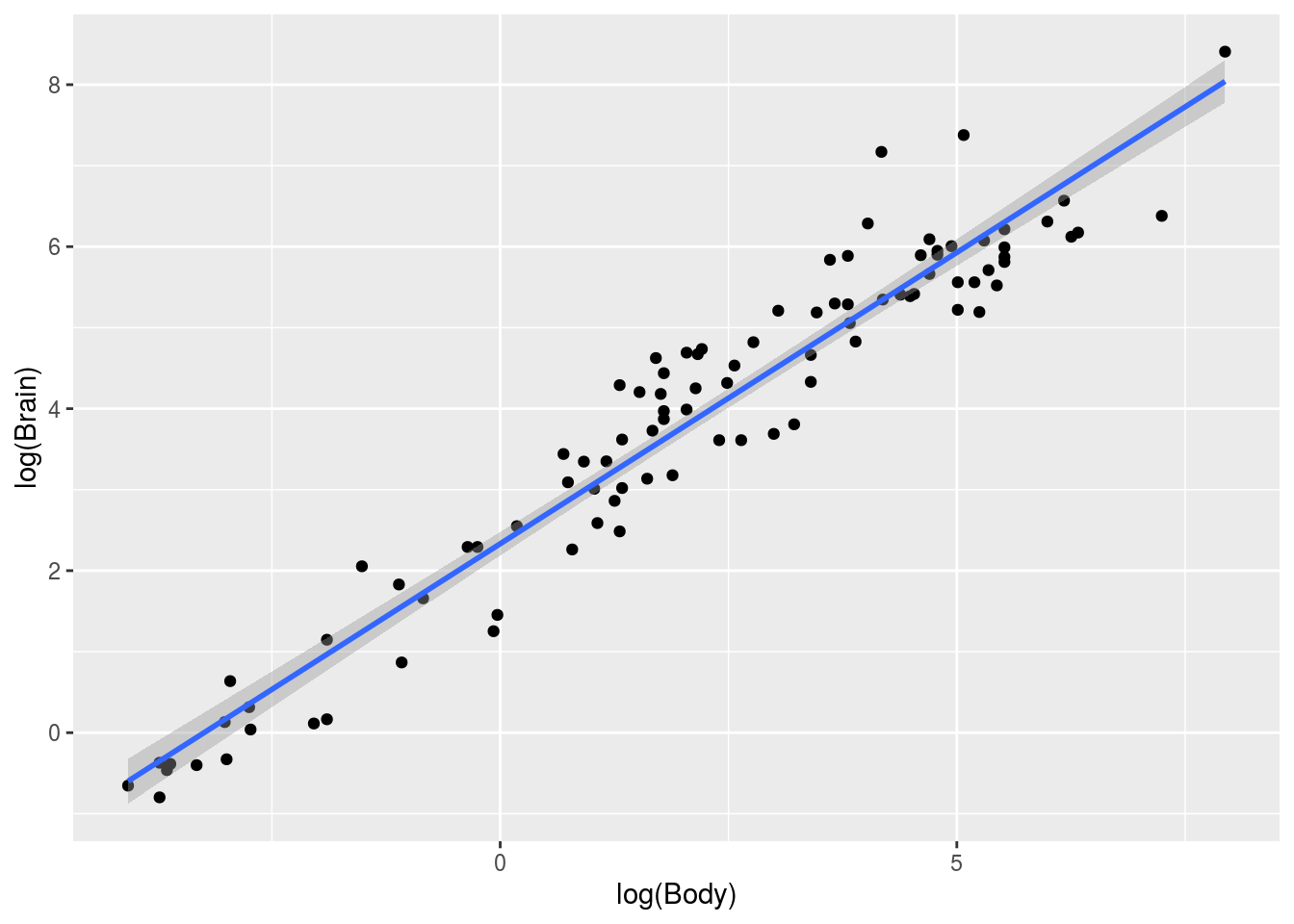Run the following to load a dataset that records various data about mammals, including brain weight. The brain weight is given in grams, the body weight in kilograms, and the gestation weight in days.

``brains <- read.csv("http://guerzhoy.princeton.edu/201s20/brains.csv")``

### Problem 1: Linear Regression

#### Part 1(a)

Suppose you want to use linear regression to investigate the relationship between brain weight and body weight. Find a way to transform the variables that would allow you to do that. (Hint: try taking the log of both variables. See Tuesday’s lecture where we explored the relationship between gdp per capita and life expectancy). Use a scatterplot to assess whether a relationship is linear.

#### Solution

A plot where we take the log of both variables works nicely.

``````ggplot(brains, mapping = aes(x = log(Body), y = log(Brain))) +
geom_point() +
geom_smooth(method = "lm")  ``````#### Part 1(b)

Produce the diagnostic plots. Display and investigate outliers, if any. (See Tuesday’s lecture on the relationship between gdp per capita and life expectancy)

Let’s now plot the diagnostic plots

#### Solutions

``````library(ggfortify)
fit <- lm(log(Brain) ~ log(Body), data = brains)
autoplot(fit)``````